Question

I have no more posting for this month, please solve these for me thanks 1. Given...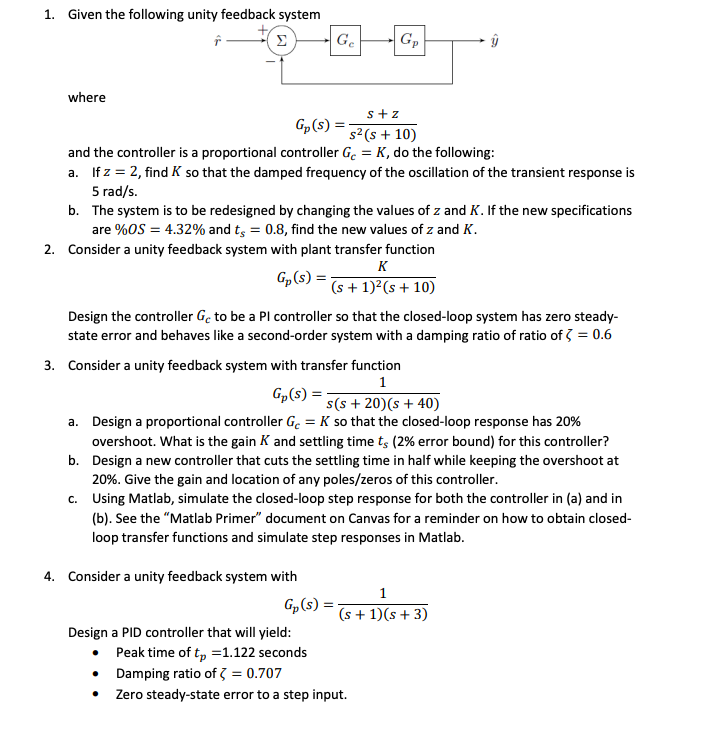I have no more posting for this month, please solve these for me thanks

1. Given the following unity feedback system where s+z s2 (s + 10) and the controller is a proportional controller Ge = K, do the following: a. If z = 2, find K so that the damped frequency of the oscillation of the transient response is 5 rad/s. b. The system is to be redesigned by changing the values of z and K. If the new specifications are %OS = 4.32% and ts = 0.8, find the new values of z and K. 2. Consider a unity feedback system with plant transfer function к G,(s) = (5 + 1)2(s + 10) Design the controller Ge to be a Pl controller so that the closed-loop system has zero steady- state error and behaves like a second-order system with a damping ratio of ratio of = 0.6 3. Consider a unity feedback system with transfer function 1 Gp(S) s(s+20) (s +40) a. Design a proportional controller Ge = K so that the closed-loop response has 20% overshoot. What is the gain K and settling time ts (2% error bound) for this controller? b. Design a new controller that cuts the settling time in half while keeping the overshoot at 20%. Give the gain and location of any poles/zeros of this controller. C. Using Matlab, simulate the closed-loop step response for both the controller in (a) and in (b). See the "Matlab Primer" document on Canvas for a reminder on how to obtain closed- loop transfer functions and simulate step responses in Matlab. 4. Consider a unity feedback system with 1 Gp(s) Gp(S) = TS + 1)(8 + 3) Design a PID controller that will yield: • Peak time of tp =1.122 seconds • Damping ratio of = 0.707 • Zero steady-state error to a step input.

We need at least 10 more requests to produce the answer.

0 / 10 have requested this problem solution

The more requests, the faster the answer.

All students who have requested the answer will be notified once they are available.

Earn Coins

Coins can be redeemed for fabulous gifts.

Similar Homework Help Questions
• Please show calculations by HAND and NOT MATLAB. The answers are here to help. Thank you Note : Ts= 4/&*wn (&=damping ratio) Skill-Assessment Exercise 9.3 PROBLEM: A unity feedback system wit...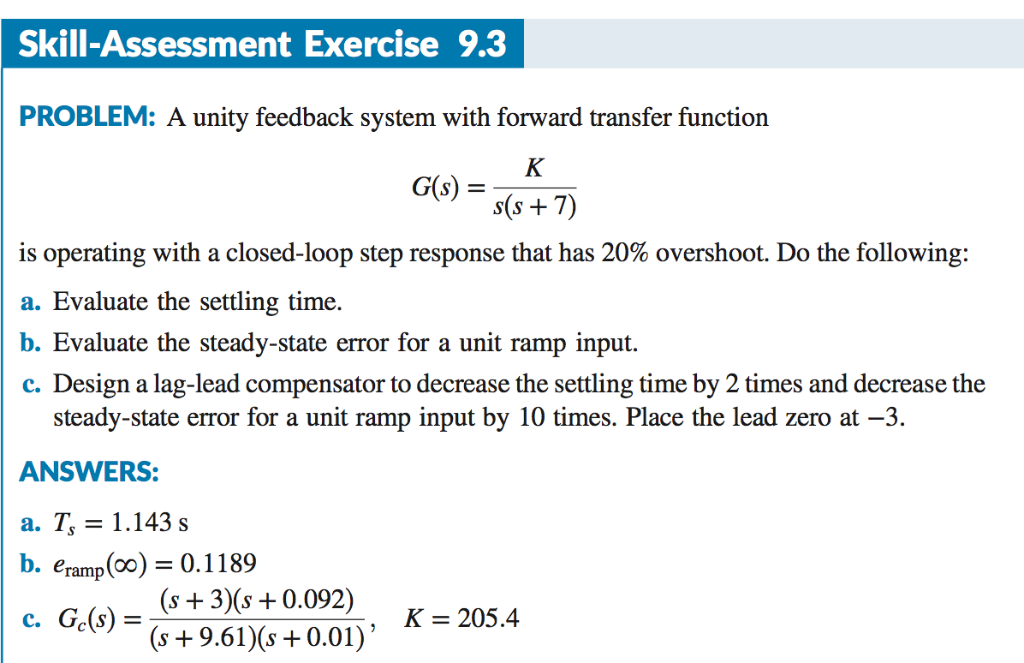Please show calculations by HAND and NOT MATLAB. The answers are here to help. Thank you Note : Ts= 4/&*wn (&=damping ratio) Skill-Assessment Exercise 9.3 PROBLEM: A unity feedback system with forward transfer function 6) s(s + is operating with a closed-loop step response that has 20% overshoot. Do the following: a. Evaluate the settling time. b. Evaluate the steady-state error for a unit ramp input. c. Design a lag-lead compensator to decrease the settling time by 2 times and...

• Consider a unity-feedback control system with a PI controller Gpr(s) and a plant G(s) in cascade. In particular, the plant transfer function is given as 2. G(s) = s+4, and the PI controller trans...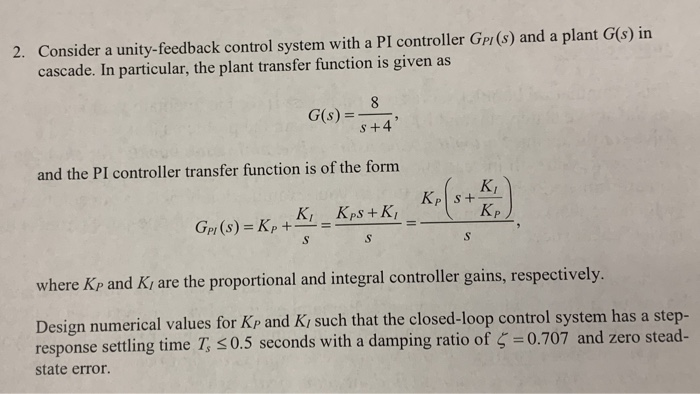Consider a unity-feedback control system with a PI controller Gpr(s) and a plant G(s) in cascade. In particular, the plant transfer function is given as 2. G(s) = s+4, and the PI controller transfer function is of the forrm KI p and Ki are the proportional and integral controller gains, respectively where K Design numerical values for Kp and Ki such that the closed-loop control system has a step- response settling time T, 0.5 seconds with a damping ratio of...

• A unity feedback system with the forward transfer function G)2)(s +5) is operating with a closed-...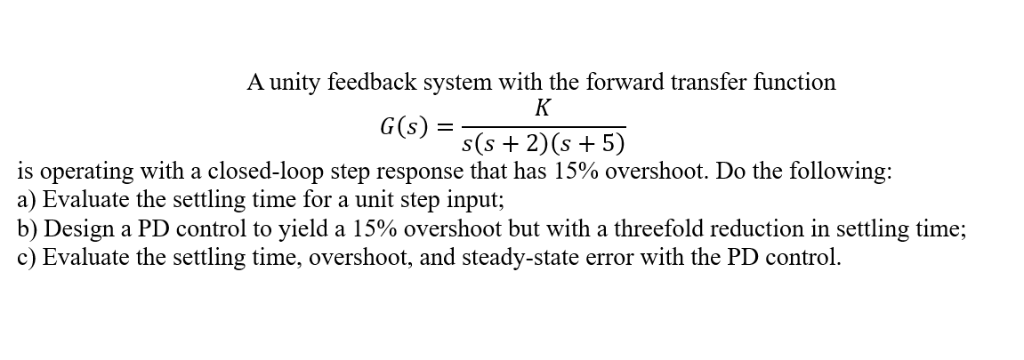A unity feedback system with the forward transfer function G)2)(s +5) is operating with a closed-loop step response that has 15% overshoot. Do the following: a) Evaluate the settling time for a unit step input; b) Design a PD control to yield a 15% overshoot but with a threefold reduction in settling time; c) Evaluate the settling time, overshoot, and steady-state error with the PD control. A unity feedback system with the forward transfer function G)2)(s +5) is operating with...

• Question #4 (25 points): Consider the open loop system that has the following transfer function 1 G(S) = 10s+ 35 Us...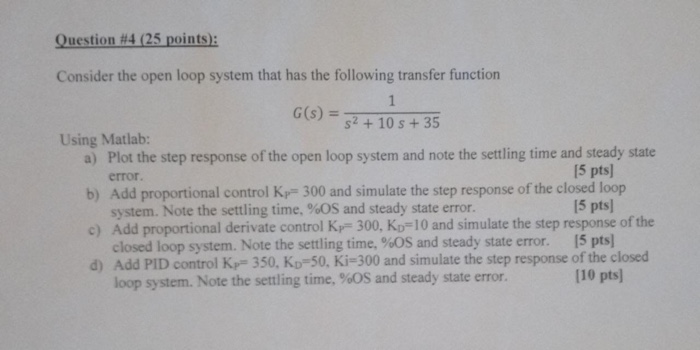Question #4 (25 points): Consider the open loop system that has the following transfer function 1 G(S) = 10s+ 35 Using Matlab: a) Plot the step response of the open loop system and note the settling time and steady state 15 pts error. b) Add proportional control K 300 and simulate the step response of the closed loop 15 pts system. Note the settling time, %OS and steady state error. c) Add proportional derivate control Kp 300, Ko 10 and...

• Q3. Consider a single loop unity feedback control system of the open loop transfer function (a) Find the range of values of the gain K and the parameter p so that: (i) The overshoot is less than 10%....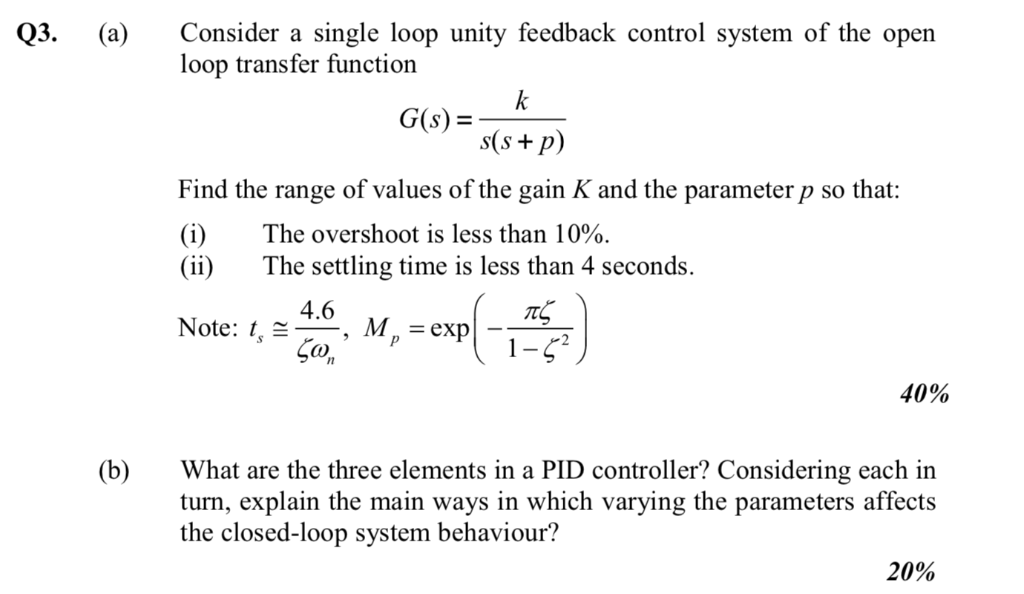Q3. Consider a single loop unity feedback control system of the open loop transfer function (a) Find the range of values of the gain K and the parameter p so that: (i) The overshoot is less than 10%. (ii)The settling time is less than 4 seconds Note: , 4.6 M. = exp CO 40% (b)What are the three elements in a PID controller? Considering each in turn, explain the main ways in which varying the parameters affects the closed-loop system...

• A unity feedback system with the forward transfer function G (s) = s(s+2)(s15) is operating with ...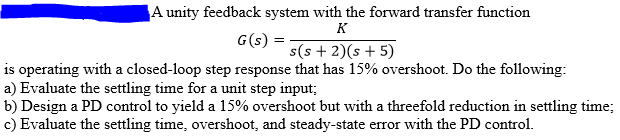A unity feedback system with the forward transfer function G (s) = s(s+2)(s15) is operating with a closed-loop step response that has 15% overshoot. Do the following: a) Evaluate the settling time for a unit step input b) Design a PD control to yield a 15% overshoot but with a threefold reduction in settling time; c) Evaluate the settling time, overshoot, and steady-state error with the PD control. A unity feedback system with the forward transfer function G (s) =...

• Problem 4. Consider the control system shown below with plant G(s) that has time con- stants...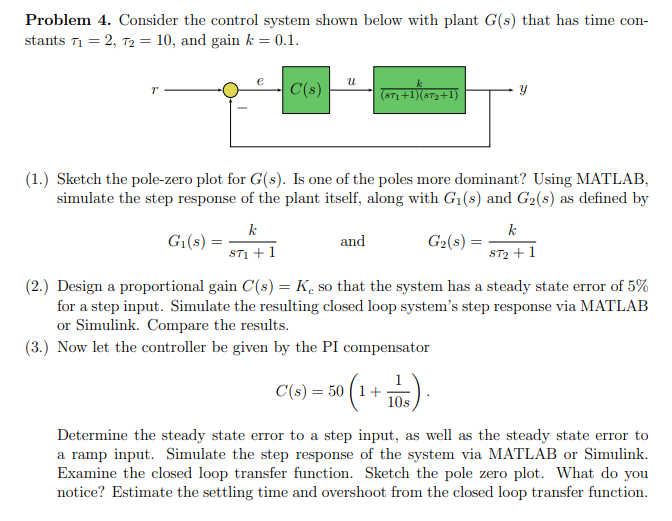Problem 4. Consider the control system shown below with plant G(s) that has time con- stants T1 = 2, T2 = 10, and gain k = 0.1. 4 673 +1679+1) (1.) Sketch the pole-zero plot for G(s). Is one of the poles more dominant? Using MATLAB, simulate the step response of the plant itself, along with G1(s) and G2(s) as defined by Gl(s) = and G2(s) = sti + 1 ST2+1 (2.) Design a proportional gain C(s) = K so...

• Problem 6 Design a PI controller to drive the step-response error to zero for the negative unity ...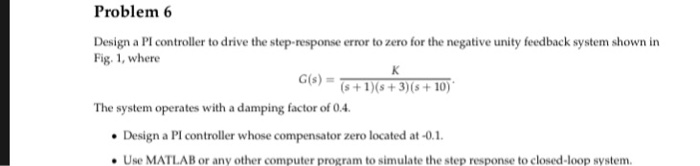Problem 6 Design a PI controller to drive the step-response error to zero for the negative unity feedback system shown in Fig. 1, where G(s) = (s+1)(s + 3)(s+ io). The system operates with a damping factor of 0.4 Design a PI controller whose compensator zero located at-0.1 Use MATLAB or any other computer program to simulate the step response to closed-loop system Problem 6 Design a PI controller to drive the step-response error to zero for the negative unity...

• Design a PI controller to drive the step-response error to zero for the negative unity feedback s...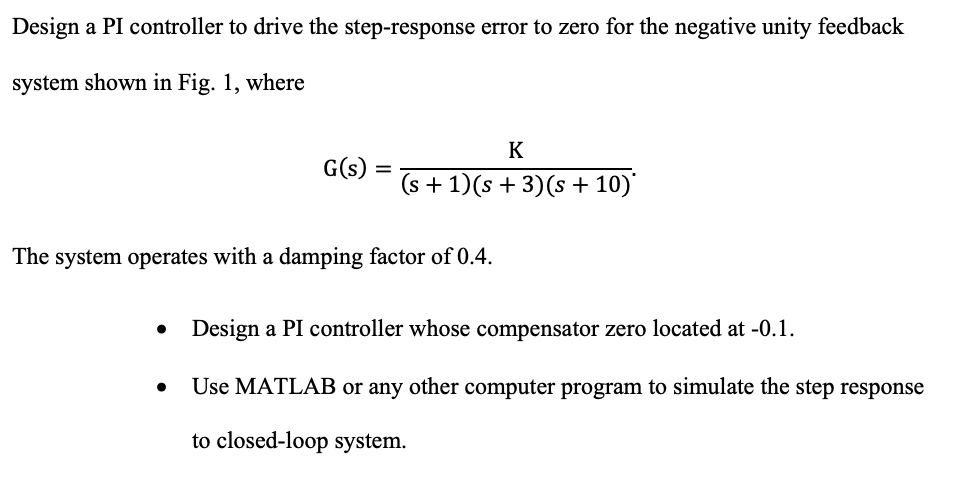Design a PI controller to drive the step-response error to zero for the negative unity feedback system shown in Fig. 1, where G(s) S+1(s+3)(s+10) The system operates with a damping factor of 0.4. * Design a PI controller whose compensator zero located at -0.1 Use MATLAB or any other computer program to simulate the step response * to closed-loop system Design a PI controller to drive the step-response error to zero for the negative unity feedback system shown in Fig....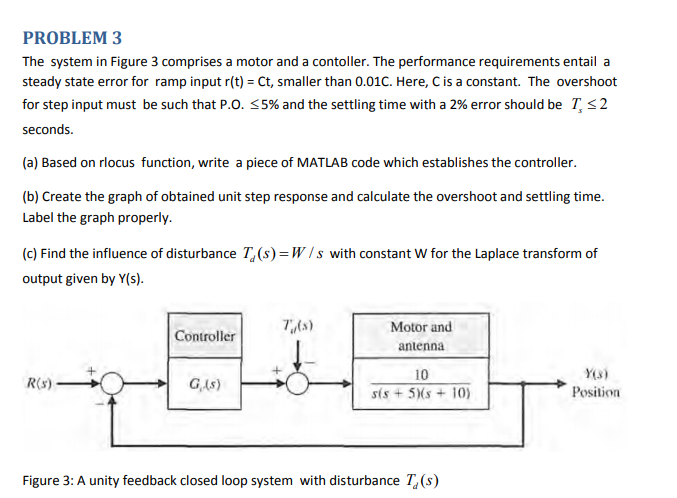PLEASE SOLVE IN MATLAB !!!!!! PLEASE SOLVE IN MATLAB !!!!!! PLEASE SOLVE IN MATLAB !!!!!! PLEASE SOLVE IN MATLAB !!!!!! PLEASE SOLVE IN MATLAB !!!!!! PLEASE SOLVE IN MATLAB !!!!!! PLEASE SOLVE IN MATLAB !!!!!! PLEASE SOLVE IN MATLAB !!!!!! PLEASE SOLVE IN MATLAB !!!!!! PROBLEM 3 The system in Figure 3 comprises a motor and a contoller. The performance requirements entail a steady state error for ramp input r(t)-Ct, smaller than 0.01C. Here, C is a constant. The overshoot...